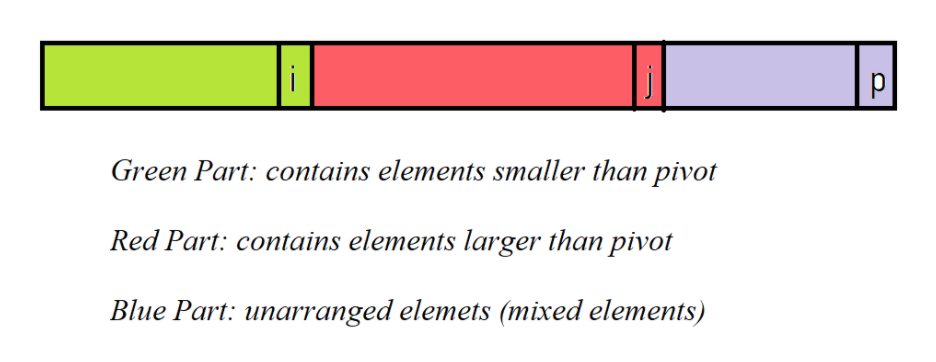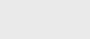1年前 (2021-12-29) |   抢沙发  153

[收起] 文章目录1. 第1部分在数组之前，数组中的所有元素都必须小于pivot。

2. 第2部分在数组之后，数组中的所有元素都必须大于pivot

## 分区是如何工作的？• `p`：枢轴元件
• `i`：标记数组绿色部分的最后一个元素
• `j`：标记数组红色部分的最后一个元素

``````partition(A[], int l, int r)
{
// select a pivot element
// right most element is selected as pivot
pivot= A[r]
// initially green and red part of arrays donot exist
i = l-1
for(j=l; j <= r; j++)
{
//comparing element with pivot
if(A[j] < pivot)
{
i++
swap A[i] and A[j]
}
}
swap A[i+1] and A[j]
return (i+1)
}``````

1. 拾取第一个元素作为轴

2. 选择最后一个元素作为轴

3. 选择随机元素作为轴心

4. 选择中间带元素作为轴

## 快速排序代码示例

``````public class QuickSort
{
public static void main(String args[])
{
int arr[] = {10, 7, 8, 9, 1, 5};
quickSort(arr, 0, arr.length-1);
for(int i=0;i<arr.length;i++)
{
System.out.print(arr[i]+" ");
}

}

static void quickSort(int arr[], int l, int r)
{
if(l<r) //check if length of subarray is greater than 1
{
// this will set pivot element in the correct position
int pi=partition(arr, l, r);
// elements before the pivot will be sorted now
quickSort(arr, l, pi-1);
// elements after the pivot will be sorted
quickSort(arr, pi+1, r);
}
}

//partition algorithm as already explained above
static int partition(int arr[], int low, int high)
{
int pivot = arr[high];
int i = (low-1); // index will follow smaller element
for (int j=low; j<high; j++)
{
// check if element at j smaller than pivot
if (arr[j] < pivot)
{
i++;

// swap arr[i] and arr[j]
int temp = arr[i];
arr[i] = arr[j];
arr[j] = temp;
}
}

// swap arr[i+1] and arr[high] (or pivot)
int temp = arr[i+1];
arr[i+1] = arr[high];
arr[high] = temp;

return i+1;
}
}``````

## 快速排序的复杂性

``F(n)=F(k)+F(n-k-1)+θ(n)``

1. 最佳情况：当枢轴正好在数组的中间时。然后将数组分成两个相等的部分。

`F（n）=2*F（n/2）+θ（n）`

2. 最坏情况：当枢轴位于阵列边缘时，即它是阵列中最大或最小的元素。

`F（n）=2*F（n-1）+θ（n）`### 注册